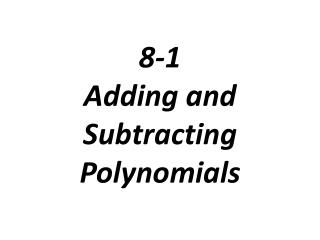# 8-1 Adding and Subtracting Polynomials - PowerPoint PPT PresentationDownload PresentationDownload Presentation## 8-1 Adding and Subtracting Polynomials

- - - - - - - - - - - - - - - - - - - - - - - - - - - E N D - - - - - - - - - - - - - - - - - - - - - - - - - - -
##### Presentation Transcript

1. 8-1 Adding and Subtracting Polynomials

2. Monomial: a real number, variable, or a product of a real number and one or more variables Example: 18 z

3. Degree of a monomial: is the sum of the exponents of its variables. The degree of a nonzero constant is 0. Zero has no degree

4. Problem 1: Finding the Degree of a Monomial What is the degree of each monomial?

5. Problem 2: Adding and Subtracting Monomials What is the sum or difference?

6. Problem 3: Classifying Polynomials Write each polynomial in standard form. What is the name of the polynomial based on its degree and number of terms?

7. Problem 4: Adding Polynomials Simplify:

8. Simplify:

9. Problem 5: Subtracting Polynomials Simplify:

10. Simplify: